WO2016121857A1 - Far-infrared lens system, optical imaging device, and digital apparatus - Google Patents

Info

Publication number
WO2016121857A1
WO2016121857A1 PCT/JP2016/052457 JP2016052457W WO2016121857A1 WO 2016121857 A1 WO2016121857 A1 WO 2016121857A1 JP 2016052457 W JP2016052457 W JP 2016052457W WO 2016121857 A1 WO2016121857 A1 WO 2016121857A1
Authority
WO
WIPO (PCT)
Prior art keywords
lens
far
infrared
lens system
surface
Prior art date
Application number
PCT/JP2016/052457
Other languages
French (fr)
Japanese (ja)
Inventor

Original Assignee
コニカミノルタ株式会社
Priority date (The priority date is an assumption and is not a legal conclusion. Google has not performed a legal analysis and makes no representation as to the accuracy of the date listed.)
Filing date
Publication date
Priority to JP2015015427 priority Critical
Priority to JP2015-015427 priority
Application filed by コニカミノルタ株式会社 filed Critical コニカミノルタ株式会社
Publication of WO2016121857A1 publication Critical patent/WO2016121857A1/en

Images

••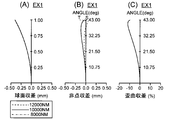•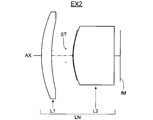•••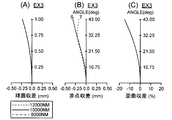•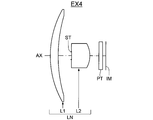•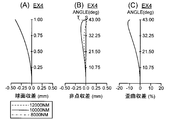•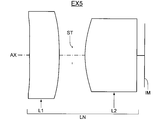•••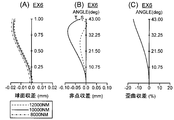•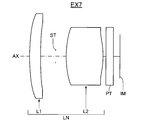••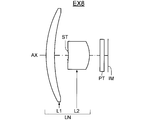•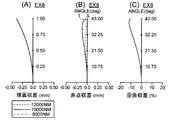•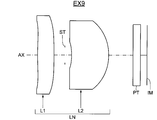•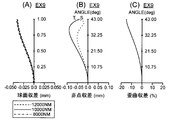•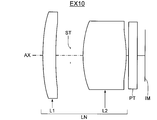•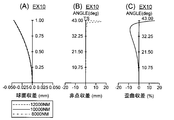•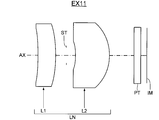•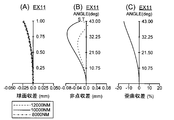•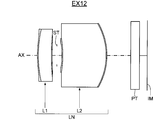••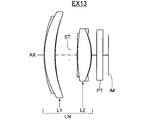•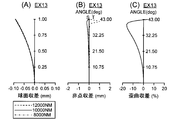••••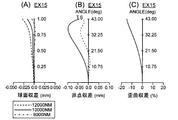•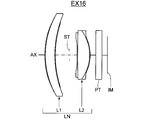•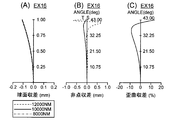•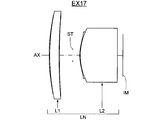••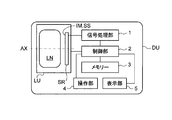Classifications

• GPHYSICS
• G02OPTICS
• G02BOPTICAL ELEMENTS, SYSTEMS, OR APPARATUS
• G02B13/00Optical objectives specially designed for the purposes specified below
• G02B13/14Optical objectives specially designed for the purposes specified below for use with infra-red or ultra-violet radiation
• GPHYSICS
• G02OPTICS
• G02BOPTICAL ELEMENTS, SYSTEMS, OR APPARATUS
• G02B13/00Optical objectives specially designed for the purposes specified below
• G02B13/001Miniaturised objectives for electronic devices, e.g. portable telephones, webcams, PDAs, small digital cameras
• G02B13/0015Miniaturised objectives for electronic devices, e.g. portable telephones, webcams, PDAs, small digital cameras characterised by the lens design
• G02B13/002Miniaturised objectives for electronic devices, e.g. portable telephones, webcams, PDAs, small digital cameras characterised by the lens design having at least one aspherical surface
• G02B13/003Miniaturised objectives for electronic devices, e.g. portable telephones, webcams, PDAs, small digital cameras characterised by the lens design having at least one aspherical surface having two lenses
• GPHYSICS
• G02OPTICS
• G02BOPTICAL ELEMENTS, SYSTEMS, OR APPARATUS
• G02B13/00Optical objectives specially designed for the purposes specified below
• G02B13/001Miniaturised objectives for electronic devices, e.g. portable telephones, webcams, PDAs, small digital cameras
• G02B13/008Miniaturised objectives for electronic devices, e.g. portable telephones, webcams, PDAs, small digital cameras designed for infrared light
• GPHYSICS
• G02OPTICS
• G02BOPTICAL ELEMENTS, SYSTEMS, OR APPARATUS
• G02B9/00Optical objectives characterised both by the number of the components and their arrangements according to their sign, i.e. + or -
• G02B9/04Optical objectives characterised both by the number of the components and their arrangements according to their sign, i.e. + or - having two components only
• G02B9/06Optical objectives characterised both by the number of the components and their arrangements according to their sign, i.e. + or - having two components only two + components
• GPHYSICS
• G02OPTICS
• G02BOPTICAL ELEMENTS, SYSTEMS, OR APPARATUS
• G02B13/00Optical objectives specially designed for the purposes specified below
• G02B13/18Optical objectives specially designed for the purposes specified below with lenses having one or more non-spherical faces, e.g. for reducing geometrical aberration

Abstract

A far-infrared lens system is used for far-infrared wavelengths, and configures two lenses comprising, in the order from an object side, a first positive lens and a second positive lens. A refractive ratio of a lens material configuring the greatest core thickness in each lens is greater than 2.0 and less than or equal to 3.9 at a wavelength of 10 µm. A conditional expression 2.50 < f1 / f < 7.40 is satisfied, where f1 represents a focal distance of a first lens L1, and f represents a focal distance of an entire far-infrared lens system LN. The half field of view ω of the far-infrared lens system is greater than 30°.

Description

Far-infrared lens system, an imaging optical device and digital equipment

The present invention, far-infrared lens system, to an imaging optical device and digital equipment. For example, far-infrared band an imaging lens system used (wavelength: 8 ~ 12 [mu] m band), the number of lenses two and less aberration correction is performed satisfactorily in particular at a greater angle than the half angle of view ω is 30 ° and has a low cost camera systems that can be used for far-infrared lens system, a far an imaging optical system a far-infrared image obtained by the infrared lens system captures the far infrared sensor, an image input with function equipped with far-infrared lens system and digital devices, the present invention relates.

With the spread of surveillance cameras and security cameras, compact far-infrared lens system at a low cost is required. Lens material used for the far-infrared lens system are the expensive than common optical glass, lens volume smaller cost is suppressed. From this viewpoint, relatively wide far-infrared lens system composed of two lenses is proposed in Patent Documents 1-4.

US2013 / 0271852 A1 JP 2013-195795 JP US2012 / 0229892 A1 US6292293 B1

In the lens system disclosed in Patent Document 1 and 4, the focal length of the first lens normalized by the focal length of the entire system is taking a positive value. When the lens system described in Patent Literature 1, the focal length of the first lens takes a small positive value, positive power is relatively strong (power: quantity defined as the reciprocal of the focal length). As a result, coma outward by positive power as a first lens occurs, since the second lens is not too aberration correction can not be obtained a good performance in the configuration of a small number of lenses. In addition, the lens back also becomes too short. When the lens system described in Patent Literature 4, the focal length of the first lens takes a positive large value, the positive power becomes weaker. When the power of the first lens is too weak, a wide-angle lens system which requires a short focal length generated large aberration too large power burden of the second lens, the performance in particular with inward coma in off-axis deteriorates.

In the lens system disclosed in the above Patent Documents 2 and 3, the focal length of the first lens normalized by the focal length of the entire system takes a less negative value, the negative power becomes stronger. Power for condensing light in the negative when the power is too strong second lens becomes stronger, and as a result, was rather deteriorate the performance.

In the lens system disclosed in the above Patent Documents 2 and 3, the back focus is longer normalized by the focal length. Further, since the brightness of the system is far-infrared lens system also affects the resolution has become bright F number is 2 or less, has a structure not to cut possible also off-axis light flux. In such a lens system, when the distance from the back focus is long image surface to the second lens is long, a high position from the optical axis of the second lens for passing the F-number light, the spherical aberration correction in the second lens the burden is increased. Moreover, since the axial beam and the off-axis light flux passes almost the same height, effective correction (correction of field curvature) of the off-axis performance it is hard to do. Thus, sufficient power may not be obtained in a system of a small number of lenses.

In the lens system disclosed in Patent Document 1, the meniscus of the relatively weak lens is arranged a concave surface facing the object side as a first lens. Meniscus level are those determined by the paraxial radius of curvature of the front and rear faces of the lens, the front radius of curvature R1, and the radius of curvature of the posterior surface R2, represented by (R1 + R2) / (R1-R2). Given the radius of curvature code be a value including, it indicates that the radius of curvature of the front and rear surfaces as the absolute value of which is large is large near the meniscus degree. Those described in Patent Document 1 is intensified weak power meniscus of a positive lens, to generate a spherical aberration and field curvature due to the positive optical power in the first lens. Although aberration is slightly smaller due to the positive power of the second lens, it is not performed positively correct aberrations in the first lens can not be sufficiently small aberrations with a small number of lenses, particularly poor performance at the wide angle lens system It has become easier to.

In the lens system disclosed in the above Patent Documents 2 and 3, the first lens has a negative lens, negative power is turned stronger this time meniscus level is low. Is generated aberration by the negative power of the first lens, there is also the effect of canceling the aberration due to the positive second lens, because the negative power is too strong, even larger through the high position from the optical axis rays in the second lens aberrations would be to generate, so that would rather worsen the performance at the wide angle lens system.

The present invention was made in view of such circumstances, and its object is far less expensive in good aberration correction is a high relative axial light flux and an off-axis light beam even with a small number of lenses two infrared lens system is to provide an imaging optical system and a digital device having the same.

To achieve the above object, the far-infrared lens system of the first invention is a lens system used in the far infrared band,
In order from the object side, a first lens having a positive power, a second lens having a positive power is composed of two lenses, the refractive index of the lens material forming the largest core thickness at each lens and 3.9 or less greater than 2.0 at the wavelength 10 [mu] m, it satisfies the following conditional expression (1), wherein the half angle of view is greater than 30 °.
2.50 <f1 / f <7.40 ... (1)
However,
f1: focal length of the first lens,
f: far-infrared focal length of the entire lens system,
It is.

Far-infrared lens system of the second aspect based on the first invention, by defining the distribution ν at a wavelength 8 ~ 12 [mu] m by the following equation (FD), the first, the largest core in each of the second lens dispersion ν of the lens material forming the thickness being greater than 100.
ν = (N10-1) / (N8-N12) ... (FD)
However,
N10: refractive index at a wavelength of 10 [mu] m,
N8: refractive index at a wavelength of 8 [mu] m,
N12: refractive index at a wavelength of 12 [mu] m,
It is.

Far-infrared lens system of the third invention, in the first or second aspect, and satisfies the following conditional expression (2).
0.11 <f2 / f1 <0.60 ... (2)
However,
f1: focal length of the first lens,
f2: focal length of the second lens,
It is.

Far-infrared lens system of the fourth invention, in the first to third any one invention, and satisfies the following conditional expression (3).
-9.40 <(R1 + R2) / (R1-R2) <3.65 ... (3)
However,
R1: the curvature of the most object side surface of the first lens radius,
R2: radius of curvature of the most image side surface of the first lens radius,
It is.

Fifth far-infrared lens system of the present invention, in any one invention of the first to fourth, and satisfies the following conditional expression (4).
0.34 <D1 / f <0.89 ... (4)
However,
D1: Total on-axis to the most image side surface of the most object side surface of the first lens core thickness,
f: far-infrared focal length of the entire lens system,
It is.

Far-infrared lens system of the sixth invention, in any one invention of the first to fifth, and satisfies the following conditional expression (5).
0.2 <LB / f <1.1 ... (5)
However,
LB: Length the distance between the most image side surface of the second lens to the image plane and equivalent air,
f: far-infrared focal length of the entire lens system,
It is.

The imaging optical apparatus of the seventh aspect of the present invention, far-converts the first to sixth far-infrared lens system according to any one of the invention, the far-infrared optical image formed on an image pickup surface into an electrical signal comprising an infrared sensor, and wherein the far-infrared lens system as far-infrared optical image of an object is formed on the imaging surface of the far-infrared sensor is provided.

Eighth digital devices invention is provided with the imaging optical device according to the seventh aspect, characterized in that the still image shooting of the subject, at least one of the functions of the moving image is added.

Ninth far infrared camera system of the present invention is characterized by having a far-infrared lens system according to any one invention of the first to sixth.

According to the present invention, since it is possible to perform an aggressive aberration correction on-axis light beam and an off-axis light beam even with a small number of lenses two, high performance and high definition by excellent aberration correction becomes possible, it becomes possible to cope with cheap far-infrared sensors that have been freshly prepared. Therefore, it is possible to achieve a far-infrared lens system of high performance even at low cost, the imaging optical system having the same. Then, the far-infrared lens system or the imaging optical system according to the present invention, night vision, thermography, mobile terminal, a camera system (e.g., a digital camera, a surveillance camera, security camera, onboard camera) by using a digital device such as a , it is possible to add a compact far-infrared image input function of high performance at low cost to digital devices.

Lens arrangement of a first embodiment (Example 1). Aberration diagram of Example 1. Lens arrangement of the second embodiment (Example 2). Aberration diagram of Example 2. Lens arrangement of the third embodiment (Example 3). Aberration diagram of Example 3. Lens arrangement of the fourth embodiment (Example 4). Aberration diagram of Example 4. Lens arrangement of a fifth embodiment (Example 5). Aberration diagram of Example 5. Sixth lens configuration diagram of an embodiment (Embodiment 6) Embodiment of. Aberration diagram of Example 6. Lens configuration diagram of an embodiment (Example 7) embodiment of the seventh. Aberration diagram of Example 7. Lens configuration diagram of an embodiment (Example 8) implementation of the eighth. Aberration diagram of Example 8. Lens configuration diagram of an embodiment (Example 9) of the implementation of the ninth. Aberration diagram of Example 9. Lens configuration diagram of an embodiment (Example 10) of the embodiment of the 10th. Aberration diagram of Example 10. Lens configuration diagram of an embodiment (Example 11) of the embodiment of the eleventh. Aberration diagram of Example 11. Lens configuration diagram of an embodiment (Example 12) of the embodiment of the 12th. Aberration diagram of Example 12. Lens configuration diagram of an embodiment (Example 13) of the embodiment of the 13th. Aberration diagram of Example 13. Lens configuration diagram of an embodiment (Example 14) of the embodiment of the 14th. Aberration diagram of Example 14. Lens configuration diagram of an embodiment (Example 15) of the embodiment of the 15th. Aberration diagram of Example 15. Lens configuration diagram of an embodiment (Example 16) of the embodiment of the 16th. Aberration diagram of Example 16. Lens configuration diagram of an embodiment (Example 17) of the embodiment of the 17th. Aberration diagram of Example 17. Schematic diagram showing a schematic configuration example of a digital device equipped with far-infrared lens system.

Hereinafter, far-infrared lens system according to the present invention, an imaging optical apparatus, a digital device or the like will be described. Far-infrared lens system according to the present invention is a lens system used in the far infrared band, in order from the object side, a first lens having a positive power, a second lens having a positive power, the 2 is composed of lenses, the refractive index of the largest core lens material comprising the thickness is not more than 3.9 greater than 2.0 at a wavelength of 10μm in each lens, and satisfies the following conditional expression (1), half angle is characterized by greater than 30 °.
2.50 <f1 / f <7.40 ... (1)
However,
f1: focal length of the first lens,
f: far-infrared focal length of the entire lens system,
It is.

First positive power, the second lens are both lens elements a mass that acts as a single lens. Therefore, not limited to a single lens made of uniform optical material, the surface of the lens core of uniform optical material, the lens core and may be those coated with a coating layer consisting of a thin layer of another material (e.g. resin material) . The lens having a coating layer include, for example, a composite lens such as composite aspherical lens. When you Ikaso the properties of the material (e.g., silicon) constituting the lens cores, it is necessary to thin the coating layer, the cemented lens is the first, not suitable as the second lens. However, it may be joined to the degree of thin material which does not impair the optical properties of the lens material composed mainly optically. That is, the material to be optically joined is assumed that the transmittance of the far-infrared band has a sufficient thickness, integral construction with the lens material becomes Lord as long as it functions as a single lens . Incidentally, Example 1 described below, a two lenses, both the first lens of Example 2 single lenses of Examples 3-11, two second lens, Examples 12-17 Example 2 the lens is a composite lens.

Further, the refractive index is the ratio of the speed of travel of light in the substance with respect to the vacuum, in the visible region is displayed with respect to the d-line (587 nm). However, since this value has no meaning in the far infrared region, often showing a refractive index for the wavelength 10μm typically. For example, the refractive index at a wavelength 10μm far infrared optical materials used conventionally, Ge = 4.004, Si = 3.418, ZnS = 2.200, a ZnSe = 2.407 like.

The material constituting the lens core and single lens, first, a lens material forming the largest core thickness in each of the second lens. In the first and second lens, and wherein the refractive index of the lens core and a single lens is 3.9 or less larger than 2.0 at a wavelength of 10 [mu] m. That is, the 3.9 following materials larger than the refractive index of 2.0 at a wavelength 10μm out of the far infrared optical material, a first, main and made lens material of the second lens.

The lens material for far infrared, germanium refractive index is greater than 3.9 (Ge) are well known in the wavelength 10 [mu] m, it is used in many infrared optical system. Because of the high refractive index is advantageous in aberration correction, material cost is very high because it is a rare mineral, has become an obstacle to be widely spread far-infrared camera. As the lens material for the smaller far-infrared than the refractive index of 2.0, sodium chloride (NaCl), inorganic crystalline materials such as potassium bromide (KBr) is known. These are inexpensive material but is disadvantageous in aberration correction for low refractive index is too, it is difficult to constitute the photographic lens system with a small number.

Of far-infrared lens material, refractive index at a wavelength 10μm as a 3.9 following typical greater than 2.0, silicon (Si, refractive index: 3.4178) and the like. Silicon, although not span the germanium refractive index, since those having a relatively high refractive index among the far-infrared lens material is sufficiently advantageous for aberration correction, an optical system of excellent performance with a small number of it is possible to. By the refractive index at a wavelength 10μm to use larger material than 2.0, it is possible to loose all curvature of the lens, Ya spherical aberration with a small lens of two in the lens system of the short focal length at the wide angle curvature such, it is possible to satisfactorily correct on-axis and off-axis aberrations. Further, by the refractive index of the wavelength 10μm uses 3.9 following materials can produce a lens system with an inexpensive material that does not contain germanium is rare material.

The first lens has a configuration in which the focal length f1 satisfies the conditional expression (1). This configuration, compared to the conventional wide-angle lens system of the general positive lens two configurations, it it is long focal length. The wide-angle lens system, the lens back with the focal length becomes shorter shortened. Although inexpensive far-infrared sensor is almost non-cooled which do not require cooling, sealing the front of the light receiving surface in the window material in order to increase the sensitivity, and between the window material and the light-receiving surface to the vacuum ing. This structure is the same even small sensors reduced screen size number of pixels, the more the lens system for a small sensor, the lens back increases must be ensured as compared with the focal length.

By setting within a defined range the focal length of the first lens normalized as described above, will be able also to secure a sufficient back focus with respect to small-sized sensor, at the same time, the first it is possible to achieve a good performance by a small number of lenses perform sufficient aberration correction lens. f1 / f a is set to be larger than the lower limit of condition (1), the lens back in the positive lens 2 lens structure sufficiently ensured, also to accommodate a small sensor with inexpensive screen size. Moreover, distortion can be prevented to coma outward or increases in the positive direction is generated. When exceeding the upper limit f1 / f of the conditional expression (1) is increased, will be the power burden of the second lens is increased, it becomes impossible to suppress the spherical aberration sufficiently small. In addition, there is a possibility that the coma will come out on the inside large.

Far infrared rays, an infrared range of mainly wavelength 7 ~ 14μm. Body temperature of humans and animals is the radiation of wavelength 8 ~ 12μm, far-infrared optical system mostly is used at a wavelength of 8 ~ 12μm. Far-infrared wavelength region of 8 ~ 12 [mu] m band is in a range capable of detecting the temperature of the material, temperature measurement, human detection in the dark, security, etc., as is often applicable.

However, at present the far-infrared camera is not widespread is or a machining difficult material or a material lens material which transmits far infrared radiation contains expensive rare material, they more than several sheets When the lens system used is because it becomes costly. Recent manufacturing techniques far infrared sensor advances in inexpensive thermopile or uncooled microbolometer like also come to be manufactured, inexpensive lens system to fit these is desired. The far-infrared lens system according to the present invention, constituted by two first and second lenses in order from the object side, by a lens system of small number of inexpensive by reducing the processing cost of the lens system it is made possible to provide a lens system.

Further, the conventional far-infrared sensor, it is almost expensive that can precisely display the temperature resolution. In such sensors, in order to sufficiently exhibit the temperature resolution, it is necessary to cool the sensor around in a refrigerant such as liquid nitrogen. Accordingly, since the space for cooling is required, the lens back relatively short tends wide-angle lens system has hardly been manufactured. However, there is a need that want to see a more wide field of view, yet is non-cooled sensor of the micro-bolometer, etc. that does not require a cooling in recent years have come to be produced at a low cost. Therefore, even in a half field angle ω is 30 ° greater than the angle of the far-infrared lens system implementation is possible. Such wide-angle high performance and both are allowed while the lens desirable condition setting in order to allow any two like will be described below.

Respect focal length of the first lens, it is desirable to satisfy the following conditional expression (1a), it is further desirable to satisfy the conditional expression (1b).
2.50 <f1 / f <6.76 ... (1a)
3.73 <f1 / f <6.01 ... (1b)
These conditional expressions (1a), (1b), the conditional expression (1) is also within the conditional range specified, it defines a further preferable condition range based on the viewpoint and the like. Thus, preferably conditional expressions (1a), more preferably by satisfying the formula (1b), can be further increased more the effect.

When the dispersion ν of a wavelength 8 ~ 12 [mu] m is defined by the following formula (FD), the first, the dispersion ν of the lens material forming the core thickness greatest in each of the second lens is larger, it is more desirable than 100.
ν = (N10-1) / (N8-N12) ... (FD)
However,
N10: refractive index at a wavelength of 10 [mu] m,
N8: refractive index at a wavelength of 8 [mu] m,
N12: refractive index at a wavelength of 12 [mu] m,
It is.

As a value representing the properties of the dispersion, the Abbe number νd at the d-line is used in visible light. The Abbe number is expressed by νd = (Nd-1) / (Nf-Nc) (proviso, Nd: refractive index at the d-line, Nf is the refractive index at the F-line, Nc is refraction at the C-line rate, it is.). However, since this value has no meaning in the far infrared region, in the far-infrared lens system, as a value representing the properties of the dispersion formula (FD): Table at ν = (N10-1) / (N8-N12) and using a value ν being. Because the difference in refractive index by color as this value ν is large is small, it comes to small dispersion. For example, the dispersion of the far-infrared optical materials which are conventionally used are, Ge = 750 or more, Si = 1860, ZnS = 23 (using the achromatic.) (Used for achromatic.) ZnSe = 57, and the like.

As described above, it is preferred values ​​of the most core thickness larger lens material dispersion ν of each lens is greater than 100. Such materials typical of is Si, is about to [nu = 1860, as described above. When using such a low-dispersion material, wavelength 8 ~ 12 [mu] m, the far-infrared lens system it is necessary to perform color correction in the wavelength 7 ~ 14 [mu] m and a wide wavelength band depending on the application, a considerable advantage in terms of chromatic aberration design is possible. Even for applications where high performance lens is required, it is a lens system of sufficient performance without any special color correction by the diffraction grating or the like can be obtained with a small number of two, a lens unit cost it can be of. Further, Si is because raw material in comparison with Ge is inexpensive, it is possible to further cost reduction.

If the variance ν is made of a material of small highly dispersed than 100, there is a possibility that the chromatic aberration correction becomes insufficient. Be suppressed to a small aberration at wavelength 10μm with a large number of non-spherical, the spot diameter becomes ten times several times the pixel pitch, becomes a state far infrared image can be acquired is blurred, to obtain a sufficient resolution it becomes difficult.

It is desirable to satisfy the following conditional expression (2).
0.11 <f2 / f1 <0.60 ... (2)
However,
f1: focal length of the first lens,
f2: focal length of the second lens,
It is.

As described above, as can be secured sufficient back focus with respect to inexpensive small screen size far infrared sensors, but defines the focal length range of the first lens by the conditional expression (1), wide-angle If the lens system is difficult to performance to be considered the ratio of the focal length of the second lens at the same time obtaining a good optical system of utility. By satisfying the conditional expression (2) defines the focal length ratio of the first lens and the second lens, the burden of aberration correction in a wide-angle lens system suitably shared by the respective lenses, the lens system of good performance it can be realized with a small number of two.

Beyond the upper limit of condition (2), the focal length of the second lens is larger than the focal length of the first lens, in order to wide-angle lens system the distance between the first lens and the second lens must close, the first lens and no longer sufficient space is obtained to place the barrel part or stop between the second lens, it becomes difficult to construct the lens system. Further, since the passage of the light beam a similar height by the first lens and the second lens, when securing the performance of the on-axis, such as spherical aberration, it is difficult to sufficiently correct curvature of field. Conversely, beyond the lower limit of condition (2), the focal length of the second lens is smaller than the focal length of the first lens, with the total length of the lens system becomes large, the second lens is in axial rays to generate a large spherical aberration, it is refracted inward strongly off-axis light beam will by generating coma aberration, it is difficult to obtain good optical performance.

It is desirable to satisfy the following conditional expression (2a), it is further desirable to satisfy the conditional expression (2b).
0.12 <f2 / f1 <0.40 ... (2a)
0.12 <f2 / f1 <0.25 ... (2b)
These conditional expressions (2a), (2b) are among the well condition range in which the conditional expression (2) is defined, and defines a further preferable condition range based on the viewpoint and the like. Thus, preferably conditional expressions (2a), more preferably by satisfying the formula (2b), can be further increased more the effect.

It is desirable to satisfy the following conditional expression (3).
-9.40 <(R1 + R2) / (R1-R2) <3.65 ... (3)
However,
R1: the curvature of the most object side surface of the first lens radius,
R2: radius of curvature of the most image side surface of the first lens radius,
It is.

The wide-angle lens system, for the angle of light rays incident on the first lens is large, the shape of the first lens is a large effect on the performance. Condition (3) of the (R1 + R2) / (R1-R2) is what is referred to as "shaping factor" indicating the shape of the one lens, the curvature of the lens front surface (the most object side surface) radius R1 and the lens rear surface ( It shows the relationship between the radius of curvature R2 of the most image side surface). Although plus or minus sign is different in the orientation of the lens surface, when both sides of the curvature radius is close, including code becomes a meniscus of strong lens, the absolute value of the shaping factor is increased, conversely, both sides of the curvature radius of the code If you are away included, becomes weak lens having the meniscus level, the absolute value of the shaping factor is reduced. The radius of curvature R1 has a value of plus convex to the object side, a first lens because it has a positive power, so that a strong enough meniscus degree takes a negative large value.

So as to satisfy the conditional expression (3), the shaping factor of the first lens is set within the prescribed range, by a little strong positive lens meniscus degree moderate, spherical aberration and curvature of field in the first lens mainly corrects the like, it is possible to improve the performance to offset the aberration due to the positive power generated in the second lens. When shaping factor increases beyond the upper limit of condition (3), the meniscus of the positive lens becomes extremely weak, since the off-axis light ray before and after the first lens is refracted greatly, out coma outside It tends to worsen sister performance. When shaping factor decreases beyond the lower limit of condition (3), the meniscus of the positive lens becomes strong, since the off-axis light rays that will pass through a higher position on the object side surface of the first lens, the curvature of field It tends to worsen the performance increase.

It is desirable to satisfy the following conditional expression (4).
0.34 <D1 / f <0.89 ... (4)
However,
D1: Total on-axis to the most image side surface of the most object side surface of the first lens core thickness,
f: far-infrared focal length of the entire lens system,
It is. Incidentally, when the i-th axial distance and di from the object side, when the first lens is a single lens, D1 = d1 (core thickness on the axis of the first lens), when the first lens is a complex lens, D1 = is d1 + d2 + d3 ... (total of the axial center thickness of the first lens).

The wide-angle lens system, the thickness of the first lens off-axis light flux is incident at a large angle greatly affects the performance, in the far-infrared lens system according to the present invention, first normalized by the focal length of the entire system 1 it is preferable to set the total core thickness of the lens within a predetermined range, the condition (4) defines the scope thereof. When the core thickness of the first lens exceeds the lower limit of condition (4) becomes smaller, the off-axis light beam in a similar height as the most image side surface of the most object side surface of the first lens, and, similar curvature it means that passes through the portion, curvature or the like generated by the first lens reaches the second lens without being sufficiently corrected, and finally with sufficient good aberration small number of lenses that two can not correct it is difficult to obtain good performance. If the total core thickness of the first lens exceeds the upper limit of condition (4) becomes larger, whose distance from the object side to the aperture increases, since the high position of the first lens is off-axis light flux passes, outwardly will by generating coma aberration, it is possible to obtain a lens system of excellent performance with a small number of lenses two becomes difficult.

It is desirable to satisfy the following conditional expression (5).
0.2 <LB / f <1.1 ... (5)
However,
LB: Length the distance between the most image side surface of the second lens to the image plane and equivalent air,
f: far-infrared focal length of the entire lens system,
It is.

When the light receiving surface size is inexpensive considering that the wide-angle lens system which can also be used for small-sized sensor, as described above, structural parts such as a cover glass even sensor size is compact and substantially the same structure since the ringing, a long back focal length is required than the screen size is small sensor. So as to satisfy the conditional expression (5), by setting the focal length at normalized lens back (the back focus) within the specified range, a large distance from the image plane (the sensor surface) to the second lens it without too, at the same time as the spherical aberration can be suppressed to a lower position of the second lens through which F-number light, it becomes possible to effectively carry out the correction of field curvature with respect to off-axis light flux. Further, it is possible to space for inserting the cover glass of the far infrared sensor also sufficiently secured.

When conditional expression (5) lenses back beyond the lower limit of the decrease becomes difficult to secure the space for the cover glass located in front of the sensor light receiving surface be reduced as much as possible an optical member, configuring the imaging lens system It becomes difficult. In this case, it is not possible to seal around the sensor light-receiving surface in a vacuum, the sensor itself heat will ride on the image as noise, there is a risk that a clear image can not be obtained. Further, conditional expression (5) lenses back beyond the upper limit of the increase, the total lens length becomes large, sufficiently correct the off-axis light beam and the elevated position coma and curvature of the outside through axis of the lens along therewith it is difficult to. As a result, it constitutes a good lens system with a small number of two difficult.

Far-infrared lens system according to the present invention is suitable as an imaging lens system of far-infrared camera system. As described above, the lens material and lens processing and the like be expensive to one of the causes of far-infrared camera is not popular. With the lens system of the simple two-lens structure described above, it is possible to process cost and the like of the lens to achieve an inexpensive lens system is suppressed.

The far-infrared lens system according to the present invention, the first, on at least one surface of the lens surface in which the second lens has, may be subjected to diffraction grating. By providing the diffraction grating, it is possible to satisfactorily perform correction such as axial chromatic aberration. The cross-sectional shape of the diffraction grating may be used in addition to step (stepped) shape or kinoform binary shape.

The far-infrared lens system according to the present invention, it is assumed the use of silicone materials used as a cover glass attached to the far infrared ray sensor may be used made of germanium. Further, in case that integrating the cover glass for the second lens and the sensor, the second lens may be used the same material as the cover glass, using a different material from the cover glass, and, it may be arranged close to the cover glass and the image plane side of the second lens to the plane.

Each configuration is the condition set as described above, by employing a combination alone or if necessary, make aggressive aberration correction even for axial rays and off-axis light beam with a small number of lenses two so that it is. Therefore, it is possible to achieve even two lenses while both the high performance and high definition wide-angle by good aberration correction, newly also available inexpensive far-infrared sensors have been manufactured Become. Therefore, it is possible to achieve a far-infrared lens system of high performance even at low cost, the imaging optical system having the same.

The far-infrared lens system or the imaging optical system, night vision, thermography, mobile terminal, a camera system (e.g., a digital camera, a surveillance camera, security camera, onboard camera) by using a digital device such as a high contrast digital devices the far infrared image input function performance becomes possible to add a compact inexpensive, it can contribute to the compactness, high performance, high functionality, and the like. As described above, since the one hand the lens material and lens processing causes the far-infrared camera is not widespread and the like that is expensive, the use of a lens system of a simple two-lens structure as far-infrared lens system Accordingly, it is possible to process cost and the like of the lens to achieve a low-cost camera system is suppressed.

Far-infrared lens system according to the present invention, far-infrared image input function digital device (such as a mobile terminal, the drive recorder, etc.) are suitable for use as an imaging optical system for a far-infrared sensor or the like for imaging this by combining, it is possible to construct a far-infrared imaging optical device that outputs as an electric signal takes in the far-infrared image of the object optically. Imaging optical apparatus is an optical apparatus constituting a main component of a camera used for taking still images and moving image shooting of a subject, for example, from the object (i.e. subject) side, far to form a far-infrared optical image of an object infrared a lens system, a far-infrared sensor (imaging device) for converting a far-infrared optical image formed by the far-infrared lens system into an electrical signal, and by providing the. Then, as far the light receiving surface of the infrared sensor (i.e. imaging surface) far-infrared optical image of the subject on are formed, by far-infrared lens system having the characteristic configuration described above are arranged, compact, low-cost in it is possible to realize a digital apparatus including the imaging optical system and it has a high performance.

Examples of far infrared image input function digital device, infrared cameras, surveillance cameras, security cameras, onboard cameras, aircraft cameras, digital cameras, video cameras, include a camera system such as a camera for video telephone, also, a personal computer , night vision devices, thermography, portable digital devices (for example, a mobile phone, a smart phone (high-function mobile phone), a tablet terminal, a portable information terminal with a small mobile computer, etc.), these peripheral devices (scanners, printers , mouse, etc.), and other digital devices (drive recorder, camera function include those equipped with internal or external to the defense equipment, etc.) and the like. As can be seen from these examples, not only it can constitute an infrared camera system by using the imaging optical system for far-infrared camera function by mounting the imaging optical system to various apparatuses, night vision function , it is possible to add a temperature measuring function. For example, it is possible to configure a digital device equipped with far infrared image input function such as a smart phone with an infrared camera.

An example of a far-infrared image input function digital device, illustrating a schematic configuration example of a digital equipment DU in schematic cross section in FIG. 35. Imaging optical apparatus LU loaded in the digital apparatus DU shown in FIG. 35, the object (i.e. subject) in order from the side, far infrared optical image (image surface) of the object far-infrared lens system to form a IM LN (AX: Light a shaft), and a, a far-infrared sensor for converting into an electrical signal (image pickup device) SR optical image IM formed on the light receiving surface (imaging surface) SS by far-infrared lens system LN. On the image plane IM side of the far-infrared lens system LN, a cover glass of the far infrared sensor SR, the optical filter or the like is disposed as required, will be located as parallel plate (not shown). When configuring an image input function digital equipment DU in this imaging optical device LU, usually becomes to place the imaging optical apparatus LU inside its body, it adopts the form needed when implementing a camera function it is possible to. For example, it is possible to configure the imaging optical apparatus LU which is unitized to be detachable or pivoted with respect to the main body of the digital device DU.

Far-infrared lens system LN includes, in order from the object side, a two single focus lens configuration made up of two lenses of the first lens and the second lens, as described above, the light-receiving surface of the far-infrared sensor SR It has been configured to form an optical image IM formed of far infrared on SS. The far-infrared sensor SR, for example, a plurality of pixels (e.g., several thousand to several hundreds of thousand pixels) has an image sensor for far infrared rays using a wavelength of about 8 ~ 12 [mu] m (Thermo sensor or the like) is used. Far-infrared lens system LN is far since infrared sensor SR of the photoelectric conversion unit in which the optical image IM of the subject on the light receiving surface SS is provided so as to be formed, an optical image formed by the far-infrared lens system LN IM is converted into an electrical signal by the far infrared sensor SR.

Specific examples of the far-infrared sensor SR, a pyroelectric sensor, a microbolometer, thermopile, and the like. Pyroelectric sensor is for using pyroelectric effect ceramic containing lead zirconate titanate or the like to the spontaneous polarization by a change in temperature, in most cases has a single light receiving surface, is an inexpensive temperature sensor. Microbolometer has a light receiving surface which is arranged two-dimensionally heat-sensitive material by microfabrication techniques such as amorphous silicon or vanadium oxide, a temperature sensor for detecting that the resistance value due to temperature rise changes. General microbolometer in current use is the number of pixels is 80 × 80,320 × 240,640 × 480 and so on. Conventionally to sufficiently exhibit the temperature resolution, but that the periphery of the sensor is cooled with liquid nitrogen or the like were most in recent years progressed manufacturing techniques, without cooling the manufactured some extent high temperature sensing capability it has been. Thermopile is a temperature sensor thermocouples was in series or sensor surface are arranged in parallel which can convert heat into electric energy, is inexpensive next to the pyroelectric sensor.

Digital devices DU, in addition to the imaging optical device LU, a signal processing unit 1, the control unit 2, memory 3, operation section 4, a display unit 5 or the like. Generated signal with far-infrared sensor SR is predetermined digital image processing and image compression processing in the signal processing unit 1 is subjected to the necessary, or is recorded in the memory 3 as a digital video signal (semiconductor memory, optical disk, etc.) optionally it is transmitted or is converted into an infrared signal or the like or via a cable to another device (e.g., mobile phone communication function). The control unit 2 is composed of a microcomputer, photographing function (still image capturing function, video recording function, etc.), control of functions such as image reproducing function; performs control of the lens moving mechanism for focusing intensively. For example, still image shooting of the subject, to perform at least one of the moving image, the control for the imaging optical device LU is performed by the control unit 2. The display unit 5 is a portion including a display such as a liquid crystal monitor, an image is displayed on the image information recorded on the image signal or the memory 3 which has been converted by the far infrared sensor SR. Operation unit 4, an operation button (e.g., release button), a moiety comprising an operating member such as an operation dial (for example, shooting mode dial), and transmits the information the operator operates input to the control unit 2.

1, 3, ..., 31, 33 respectively show embodiments of the first to seventeenth far-infrared lens system LN in an infinity in-focus state in the optical section. Far-infrared lens system LN embodiment of the first to seventeenth, in order from the object side, a first lens L1 having positive power, a second lens L2 having positive power, are made of. The first, in the embodiment of the third to 11, the first lens L1 and second lens L2 are both a single lens. In the second embodiment, the first lens L1 is a single lens, the second lens L2 is a compound lens. In the embodiment of the twelfth to seventeenth, the first lens L1 and second lens L2 are both a compound lens. Compound lens, a relatively thin with a coating layer made of a resin material, the entire lens cores made of an inorganic material (up to the edge portion of the lens) is in the configuration covered, the effective diameter from the effective area (optical axis AX coating layer other than the position range up) because it does not affect the optical performance, the coating layer other than the effective area in the lens configuration diagram are not shown.

First, third, fourth, sixth to 13, in operation 15, the sixteenth embodiment, which corresponds to the protective cover glass of the far-infrared sensor SR on the image surface IM side of each far-infrared lens system LN parallel flat plate PT is arranged. Second, fifth and 14, the seventeenth embodiment, a protective cover glass of the second lens L2 and the far infrared sensor SR are integrated.

Hereinafter, the configuration of the far-infrared lens system embodying the present invention is described more specifically to their construction data of Examples. Here mentioned Examples 1 to 17 (EX1 - 17) are numerical examples corresponding respectively to the embodiment of the first to 17 described above, a lens configuration diagram showing an embodiment of the first to seventeenth (FIGS. 1, 3, ..., 33) shows lens cross-sectional shape of the corresponding examples 1-17, the optical configuration of the lens arrangement and the like, respectively.

The construction data of the embodiment, as the surface data, in order from the left column, the surface number i (OB: the object plane, ST: stop surface, IM: an image surface), the radius of curvature r in the near-axis (mm), the axial surface distance d (mm), the design wavelength .lambda.0: refractive index N10 of at 10 [mu] m, and shows the dispersion ν of a wavelength 8 ~ 12 [mu] m (without: air).

Surface to surface number i * is attached is non-spherical, the surface shape is defined by the local orthogonal coordinate system with its origin at the surface vertex (x, y, z) below employing the (AS) that. Aspherical data, indicating the aspherical coefficients and the like. Incidentally, the coefficient of the term no notation in the aspherical surface data of the embodiment is 0, and E-n = × 10 -n for all data.
z = (c · h 2) / [1 + √ {1- (1 + K) · c 2 · h 2}] + Σ (Aj · h j) ... (AS)
However,
h: z-axis (optical axis AX) height in a direction perpendicular to (h 2 = x 2 + y 2),
z: sag amount in the optical axis AX direction at the height h (relative to the vertex),
c: the curvature (reciprocal of a paraxial curvature radius r) at the surface vertex,
K: conic constant,
Aj: j-th order aspherical coefficient, (sigma represents ∞ following summation from fourth order for j.)
It is.

As the refractive index and dispersion data of the optical material constituting each lens, the refractive index N10 of the wavelength 10 [mu] m, dispersion [nu = the (N10-1) / (N8-N12) at a wavelength 8 ~ 12 [mu] m, the following show. Incidentally, the parallel flat plate PT in front of the image plane IM is a silicon protective plate of far-infrared sensor SR (cover glass).
Silicon (Si) ... N10 = 3.4178, ν = 1860
Polyethylene ... N10 = 1.5226, ν = 15.10
Fluorine-based resin ... N10 = 1.6700, ν = 22.33

As various data (specification), the design wavelength .lambda.0 (nm), the entire system of focal length f (mm), F number (FNO), (distance from first lens surface to the image plane IM, mm) total length TL and half angle It shows the ω (°). Also shows a conditional expression corresponding values ​​and related data for each example in Table 1.

2, 4, 6, ..., 32, 34 are aberration diagrams respectively corresponding to Examples 1 ~ 17 (EX1 ~ 17), (A) shows spherical aberration diagrams, (B) non astigmatism diagrams, (C) is a distortion diagram. Since the far-infrared sensor SR that the lens system is intended varies depending embodiment, the aberration of the scale sensor pitch, such that the lens system are (microbolometer become a combined performance of 25 [mu] m, 17 .mu.m, 12 [mu] m and the like, thermopile the sensor pitch and has a 32μm and the like.).

Spherical aberration diagram (A) is a spherical aberration at the design wavelength (Evaluation wavelength) 10000 nm indicated by a solid line, the spherical aberration at the wavelength 8000nm shown by the dashed line, the spherical aberration at the wavelength 12000nm indicated by a broken line, the paraxial image plane represents in deviation amount of the optical axis AX direction (mm) from the vertical axis represents a normalized value (i.e. the relative pupil height) the height of incidence on the pupil at its maximum height. In the astigmatism diagram (B), the broken line T is tangential image surface at the design wavelength of 10000 nm, the sagittal image plane in a solid line S is a designed wavelength 10000 nm, in the amount of deviation of the optical axis AX from the paraxial image surface along (mm) it represents, and the vertical axis represents the half angle of view ω (aNGLE, °). In each distortion diagram (C), the horizontal axis represents the distortion (%) at a design wavelength 10000 nm, the ordinate represents the half angle omega (ANGLE, °). The maximum value of the half angle ω corresponds to the maximum image height Y '(half the diagonal length of the sensing surface SS of the far-infrared sensor SR) in the image plane IM.

Far-infrared lens system LN of Example 1 (EX1) (Fig. 1) includes, in order from the object side, a first lens L1 having positive power, an aperture stop ST, a second lens L2 having a positive power, it is composed of there. When viewed each lens surface shape of the paraxial, the first lens L1 is a positive meniscus lens element convex to the object side, the second lens L2 is a positive meniscus lens element convex to the image side. Parallel flat plate PT constituting the sixth surface and the seventh surface is a protective cover glass attached to the far infrared sensor SR.

Far-infrared lens system LN of Example 2 (EX2) (FIG. 3) includes, in order from the object side, a first lens L1 having positive power, an aperture stop ST and a second lens having a positive power having a coating layer on the object side and L2, and a. When viewed each lens surface shape of the paraxial, the first lens L1 is a positive meniscus lens element convex to the object side, the second lens L2 is a positive meniscus lens element convex to the object side. Most object side surface of the second lens L2 are aspherical. The second lens L2 constituting the fourth surface to the sixth surface, a cover glass for far-infrared sensor SR are integrated.

Far-infrared lens system LN of Example 3 (EX3) (Fig. 5) includes, in order from the object side, a first lens L1 having positive power, an aperture stop ST, a second lens L2 having a positive power, it is composed of there. When viewed each lens surface shape of the paraxial, the first lens L1 is a positive meniscus lens element convex to the object side, the second lens L2 is a positive meniscus lens element convex to the image side. Parallel flat plate PT constituting the sixth surface and the seventh surface is a protective cover glass attached to the far infrared sensor SR.

Far-infrared lens system LN in Example 4 (EX4) (FIG. 7) includes, in order from the object side, a first lens L1 having positive power, an aperture stop ST, a second lens L2 having a positive power, it is composed of there. When viewed each lens surface shape of the paraxial, the first lens L1 is a positive meniscus lens element convex to the object side, the second lens L2 is a positive meniscus lens element convex to the image side. Parallel flat plate PT constituting the sixth surface and the seventh surface is a protective cover glass attached to the far infrared sensor SR.

Far-infrared lens system LN of Example 5 (EX5) (Fig. 9) includes, in order from the object side, a first lens L1 having positive power, an aperture stop ST, a second lens L2 having a positive power, it is composed of there. When viewed each lens surface shape of the paraxial, the first lens L1 is a positive lens of biconvex, second lens L2 is a positive plano-convex lens having a convex surface facing the object side. Sided the object side surface of the second lens L2 of the first lens L1 is an aspherical surface. The second lens L2 constituting the fourth surface and the fifth surface, cover glass for far-infrared sensor SR are integrated.

Far-infrared lens system LN of Example 6 (EX6) (FIG. 11) includes, in order from the object side, a first lens L1 having positive power, an aperture stop ST, a second lens L2 having a positive power, it is composed of there. When viewed each lens surface shape of the paraxial, the first lens L1 is a positive meniscus lens element convex to the object side, the second lens L2 is a positive meniscus lens element convex to the image side. Both surfaces both surfaces of the second lens L2 of the first lens L1 is an aspherical surface. Parallel flat plate PT constituting the sixth surface and the seventh surface is a protective cover glass attached to the far infrared sensor SR.

Far-infrared lens system LN of Example 7 (EX7) (FIG. 13) includes, in order from the object side, a first lens L1 having positive power, an aperture stop ST, a second lens L2 having a positive power, it is composed of there. When viewed each lens surface shape of the paraxial, the first lens L1 is a positive meniscus lens element convex to the object side, the second lens L2 is a biconvex positive lens. Both surfaces both surfaces of the second lens L2 of the first lens L1 is an aspherical surface. Parallel flat plate PT constituting the sixth surface and the seventh surface is a protective cover glass attached to the far infrared sensor SR.

Far-infrared lens system LN of Example 8 (EX8) (FIG. 15) includes, in order from the object side, a first lens L1 having positive power, an aperture stop ST, a second lens L2 having a positive power, it is composed of there. When viewed each lens surface shape of the paraxial, the first lens L1 is a positive meniscus lens element convex to the object side, the second lens L2 is a positive meniscus lens element convex to the image side. Parallel flat plate PT constituting the sixth surface and the seventh surface is a protective cover glass attached to the far infrared sensor SR.

Far-infrared lens system LN of Example 9 (EX9) (FIG. 17) includes, in order from the object side, a first lens L1 having positive power, an aperture stop ST, a second lens L2 having a positive power, it is composed of there. When viewed each lens surface shape of the paraxial first lens L1 is a positive lens of biconvex, a second lens L2 is a positive meniscus lens element convex to the image side. Both surfaces both surfaces of the second lens L2 of the first lens L1 is an aspherical surface. Parallel flat plate PT constituting the sixth surface and the seventh surface is a protective cover glass attached to the far infrared sensor SR.

Far-infrared lens system LN of Example 10 (EX10) (FIG. 19) includes, in order from the object side, a first lens L1 having positive power, an aperture stop ST, a second lens L2 having a positive power, it is composed of there. When viewed each lens surface shape of the paraxial, the first lens L1 is a positive meniscus lens element convex to the object side, the second lens L2 is a biconvex positive lens. Both surfaces both surfaces of the second lens L2 of the first lens L1 is an aspherical surface. Parallel flat plate PT constituting the sixth surface and the seventh surface is a protective cover glass attached to the far infrared sensor SR.

Far-infrared lens system LN of Example 11 (EX11) (FIG. 21) includes, in order from the object side, a first lens L1 having positive power, an aperture stop ST, a second lens L2 having a positive power, it is composed of there. When viewed each lens surface shape of the paraxial first lens L1 is a positive meniscus lens element convex to the image side, the second lens L2 is a positive meniscus lens element convex to the image side. Both surfaces both surfaces of the second lens L2 of the first lens L1 is an aspherical surface. Parallel flat plate PT constituting the sixth surface and the seventh surface is a protective cover glass attached to the far infrared sensor SR.

Far-infrared lens system LN of Example 12 (EX12) (FIG. 23) includes, in order from the object side, a first lens L1 having a positive power having a coating layer on both sides, an aperture stop ST, positive having a coating layer on both surfaces a second lens L2 of the power, and a. When viewed each lens surface shape of the paraxial, the first lens L1 is a positive meniscus lens element convex to the object side, the second lens L2 is a positive meniscus lens element convex to the image side. Both surfaces both surfaces of the second lens L2 of the first lens L1 is an aspherical surface. Parallel flat plate PT constituting the tenth surface and the eleventh surface is a protective cover glass attached to the far infrared sensor SR.

Far-infrared lens system LN of Example 13 (EX13) (FIG. 25) includes, in order from the object side, a first lens L1 having a positive power having a coating layer on both sides, an aperture stop ST, positive having a coating layer on both surfaces a second lens L2 of the power, and a. When viewed each lens surface shape of the paraxial, the first lens L1 is a positive meniscus lens element convex to the object side, the second lens L2 is a biconvex positive lens. Both surfaces both surfaces of the second lens L2 of the first lens L1 is an aspherical surface. Parallel flat plate PT constituting the tenth surface and the eleventh surface is a protective cover glass attached to the far infrared sensor SR.

Far-infrared lens system LN of Example 14 (EX14) (FIG. 27) has in order from the object side, a first lens L1 having a positive power having a coating layer on both sides, an aperture stop ST, a coating layer on the object side surface a second lens L2 having a positive power, and a. When viewed each lens surface shape of the paraxial first lens L1 is a positive lens of biconvex, second lens L2 is a positive meniscus lens element convex to the object side. Sided the object side surface of the second lens L2 of the first lens L1 is an aspherical surface. The second lens L2 constituting the sixth surface to the eighth surface, a cover glass for far-infrared sensor SR are integrated.

Far-infrared lens system LN of Example 15 (EX15) (FIG. 29) includes, in order from the object side, a first lens L1 having a positive power having a coating layer on both sides, an aperture stop ST, positive having a coating layer on both surfaces a second lens L2 of the power, and a. When viewed each lens surface shape of the paraxial, the first lens L1 is a positive meniscus lens element convex to the object side, the second lens L2 is a positive meniscus lens element convex to the image side. Both surfaces both surfaces of the second lens L2 of the first lens L1 is an aspherical surface. Parallel flat plate PT constituting the tenth surface and the eleventh surface is a protective cover glass attached to the far infrared sensor SR.

Far-infrared lens system LN of Example 16 (EX16) (FIG. 31) includes, in order from the object side, a first lens L1 having a positive power having a coating layer on both sides, an aperture stop ST, positive having a coating layer on both surfaces a second lens L2 of the power, and a. When viewed each lens surface shape of the paraxial, the first lens L1 is a positive meniscus lens element convex to the object side, the second lens L2 is a biconvex positive lens. Both surfaces both surfaces of the second lens L2 of the first lens L1 is an aspherical surface. Parallel flat plate PT constituting the tenth surface and the eleventh surface is a protective cover glass attached to the far infrared sensor SR.

Far-infrared lens system LN of Example 17 (EX17) (FIG. 33) has in order from the object side, a first lens L1 having a positive power having a coating layer on both sides, an aperture stop ST, a coating layer on the object side surface a second lens L2 having a positive power, and a. When viewed each lens surface shape of the paraxial, the first lens L1 is a positive lens of biconvex, second lens L2 is a positive plano-convex lens having a convex surface facing the object side. Sided the object side surface of the second lens L2 of the first lens L1 is an aspherical surface. The second lens L2 constituting the sixth surface to the eighth surface, a cover glass for far-infrared sensor SR are integrated.

Example 1
Unit: mm
Surface data i r d N10 ν
OB INFINITY INFINITY
1 27.49962 2.389440 3.4178 1860
2 41.17168 3.929118
3 (ST) INFINITY 0.178644
4 -14.13077 5.000000 3.4178 1860
5 -6.66742 2.500000
6 INFINITY 1.000000 3.4178 1860
7 INFINITY 0.900000
IM INFINITY 0.000000

Various types of data λ0 10000.0nm
f 4.1270
FNO 1.8000
TL 14.9972
ω 43.0000 °

Example 2
Unit: mm
Surface data i r d N10 ν
OB INFINITY INFINITY
1 15.19286 1.500000 3.4178 1860
2 21.45350 2.112804
3 (ST) INFINITY 1.000000
4 * 7.47915 0.100000 1.5226 15.10
5 10.15462 6.000000 3.4178 1860
6 1.0E15 0.900000
IM INFINITY 0.000000

Various types of data λ0 10000.0nm
f 4.0287
FNO 1.8000
TL 10.7128
ω 43.0000 °

Non-spherical data aspherical: i = 4 *
K = 0.000000
A4 = -0.192121E-02
A6 = 0.000000E + 00
A8 = 0.000000E + 00
A10 = 0.000000E + 00

Example 3
Unit: mm
Surface data i r d N10 ν
OB INFINITY INFINITY
1 13.11422 1.500000 3.4178 1860
2 16.49414 2.006408
3 (ST) INFINITY 1.043581
4 -72.07863 2.590280 3.4178 1860
5 -8.85877 1.795882
6 INFINITY 1.000000 3.4178 1860
7 INFINITY 0.900000
IM INFINITY 0.000000

Various types of data λ0 10000.0nm
f 4.3769
FNO 1.8000
TL 9.9362
ω 43.0000 °

Example 4
Unit: mm
Surface data i r d N10 ν
OB INFINITY INFINITY
1 25.38763 2.176300 3.4178 1860
2 38.15760 3.547059
3 (ST) INFINITY 0.188329
4 -14.22854 5.000000 3.4178 1860
5 -6.73748 2.500000
6 INFINITY 1.000000 3.4178 1860
7 INFINITY 0.900000
IM INFINITY 0.000000

Various types of data λ0 10000.0nm
f 4.1471
FNO 1.8000
TL 14.4117
ω 43.0000 °

Example 5
Unit: mm
Surface data i r d N10 ν
OB INFINITY INFINITY
1 * 77.48277 3.457975 3.4178 1860
2 * -38.26964 1.500000
3 (ST) INFINITY 1.490901
4 * 10.20600 6.000000 3.4178 1860
5 INFINITY 0.900000
IM INFINITY 0.000000

Various types of data λ0 10000.0nm
f 3.9010
FNO 1.8000
TL 12.4489
ω 43.0000 °

Non-spherical data aspherical: i = 1 *
K = 12.752963
A4 = -0.682344E-03
A6 = 0.000000E + 00
A8 = 0.000000E + 00
A10 = 0.000000E + 00

Non-spherical data aspherical: i = 2 *
K = 41.419433
A4 = -0.441651E-03
A6 = 0.755177E-05
A8 = 0.000000E + 00
A10 = 0.000000E + 00

Non-spherical data aspherical: i = 4 *
K = -1.527898
A4 = -0.254883E-03
A6 = 0.000000E + 00
A8 = 0.000000E + 00
A10 = 0.000000E + 00

Example 6
Unit: mm
Surface data i r d N10 ν
OB INFINITY INFINITY
1 * 23.79133 1.500000 3.4178 1860
2 * 140.25331 0.632176
3 (ST) INFINITY 1.129165
4 * -8.95626 4.918622 3.4178 1860
5 * -6.59171 3.047744
6 INFINITY 1.000000 3.4178 1860
7 INFINITY 0.900000
IM INFINITY 0.000000

Various types of data λ0 10000.0nm
f 4.2924
FNO 1.8000
TL 12.2277
ω 43.0000 °

Non-spherical data aspherical: i = 1 *
K = -50.000000
A4 = -0.641911E-03
A6 = -0.575438E-04
A8 = 0.000000E + 00
A10 = 0.000000E + 00

Non-spherical data aspherical: i = 2 *
K = 50.000000
A4 = -0.148514E-02
A6 = -0.155208E-04
A8 = 0.000000E + 00
A10 = 0.000000E + 00

Non-spherical data aspherical: i = 4 *
K = 6.372835
A4 = -0.340632E-02
A6 = -0.525122E-03
A8 = 0.000000E + 00
A10 = 0.000000E + 00

Non-spherical data aspherical: i = 5 *
K = -0.128600
A4 = -0.313785E-03
A6 = -0.914653E-05
A8 = 0.000000E + 00
A10 = 0.000000E + 00

Example 7
Unit: mm
Surface data i r d N10 ν
OB INFINITY INFINITY
1 * 28.12955 1.500000 3.4178 1860
2 * 60.79737 2.038139
3 (ST) INFINITY 1.421250
4 * 13.13338 5.000000 3.4178 1860
5 * -10.29980 0.389890
6 INFINITY 1.000000 3.4178 1860
7 INFINITY 0.900000
IM INFINITY 0.000000

Various types of data λ0 10000.0nm
f 3.1761
FNO 1.8000
TL 11.3493
ω 43.0000 °

Non-spherical data aspherical: i = 1 *
K = 4.570682
A4 = -0.529627E-04
A6 = 0.893239E-05
A8 = -0.448333E-08
A10 = 0.000000E + 00

Non-spherical data aspherical: i = 2 *
K = 47.714661
A4 = 0.973790E-05
A6 = 0.122833E-04
A8 = 0.000000E + 00
A10 = 0.000000E + 00

Non-spherical data aspherical: i = 4 *
K = 8.835246
A4 = -0.822041E-03
A6 = 0.400491E-04
A8 = -0.258298E-05
A10 = 0.000000E + 00

Non-spherical data aspherical: i = 5 *
K = -50.000000
A4 = -0.296185E-02
A6 = 0.246764E-03
A8 = 0.997977E-06
A10 = 0.000000E + 00

Example 8
Unit: mm
Surface data i r d N10 ν
OB INFINITY INFINITY
1 22.65998 2.006645 3.4178 1860
2 33.99470 3.086700
3 (ST) INFINITY 0.187460
4 -14.06617 5.000000 3.4178 1860
5 -6.78391 2.500000
6 INFINITY 1.000000 3.4178 1860
7 INFINITY 0.900000
IM INFINITY 0.000000

Various types of data λ0 10000.0nm
f 4.1634
FNO 1.8000
TL 13.7808
ω 43.0000 °

Example 9
Unit: mm
Surface data i r d N10 ν
OB INFINITY INFINITY
1 * 43.76343 2.221565 3.4178 1860
2 * -206.24886 1.472347
3 (ST) INFINITY 0.901371
4 * -8.38086 5.000000 3.4178 1860
5 * -6.35757 3.263241
6 INFINITY 1.000000 3.4178 1860
7 INFINITY 0.900000
IM INFINITY 0.000000

Various types of data λ0 10000.0nm
f 4.2933
FNO 1.8000
TL 13.8585
ω 43.0000 °

Non-spherical data aspherical: i = 1 *
K = -50.000000
A4 = -0.490031E-03
A6 = -0.135950E-04
A8 = 0.000000E + 00
A10 = 0.000000E + 00

Non-spherical data aspherical: i = 2 *
K = -50.000000
A4 = -0.809774E-03
A6 = 0.200388E-05
A8 = 0.000000E + 00
A10 = 0.000000E + 00

Non-spherical data aspherical: i = 4 *
K = 11.565136
A4 = -0.253542E-02
A6 = -0.599919E-03
A8 = 0.000000E + 00
A10 = 0.000000E + 00

Non-spherical data aspherical: i = 5 *
K = 0.208177
A4 = -0.158079E-03
A6 = -0.645216E-05
A8 = 0.000000E + 00
A10 = 0.000000E + 00

Example 10
Unit: mm
Surface data i r d N10 ν
OB INFINITY INFINITY
1 * 24.39058 1.500000 3.4178 1860
2 * 50.47864 1.708722
3 (ST) INFINITY 1.463890
4 * 11.95186 5.000000 3.4178 1860
5 * -11.29497 0.271594
6 INFINITY 1.000000 3.4178 1860
7 INFINITY 0.900000
IM INFINITY 0.000000

Various types of data λ0 10000.0nm
f 3.1791
FNO 1.8000
TL 10.9442
ω 43.0000 °

Non-spherical data aspherical: i = 1 *
K = 6.112421
A4 = -0.255356E-04
A6 = 0.852556E-05
A8 = 0.271040E-08
A10 = 0.000000E + 00

Non-spherical data aspherical: i = 2 *
K = 50.000000
A4 = 0.859158E-04
A6 = 0.116900E-04
A8 = 0.000000E + 00
A10 = 0.000000E + 00

Non-spherical data aspherical: i = 4 *
K = 5.630439
A4 = -0.379398E-03
A6 = -0.168516E-04
A8 = 0.582155E-06
A10 = 0.000000E + 00

Non-spherical data aspherical: i = 5 *
K = -50.000000
A4 = -0.147977E-02
A6 = 0.864720E-04
A8 = 0.889825E-05
A10 = 0.000000E + 00

Example 11
Unit: mm
Surface data i r d N10 ν
OB INFINITY INFINITY
1 * -33.26351 2.607340 3.4178 1860
2 * -18.95000 1.552262
3 (ST) INFINITY 1.350176
4 * -9.20889 5.000000 3.4178 1860
5 * -6.76124 3.590222
6 INFINITY 1.000000 3.4178 1860
7 INFINITY 0.900000
IM INFINITY 0.000000

Various types of data λ0 10000.0nm
f 4.2912
FNO 1.8000
TL 15.1000
ω 43.0000 °

Non-spherical data aspherical: i = 1 *
K = -50.000000
A4 = -0.216985E-03
A6 = -0.154562E-04
A8 = 0.000000E + 00
A10 = 0.000000E + 00

Non-spherical data aspherical: i = 2 *
K = -50.000000
A4 = -0.634781E-03
A6 = 0.309010E-06
A8 = 0.000000E + 00
A10 = 0.000000E + 00

Non-spherical data aspherical: i = 4 *
K = 7.127641
A4 = -0.231988E-02
A6 = -0.342624E-03
A8 = 0.000000E + 00
A10 = 0.000000E + 00

Non-spherical data aspherical: i = 5 *
K = 0.073038
A4 = -0.152926E-03
A6 = -0.573821E-05
A8 = 0.000000E + 00
A10 = 0.000000E + 00

Example 12
Unit: mm
Surface data i r d N10 ν
OB INFINITY INFINITY
1 * 14.22191 0.100000 1.5226 15.10
2 14.12191 1.500000 3.4178 1860
3 26.10156 0.100000 1.5226 15.10
4 * 26.00156 0.545922
5 (ST) INFINITY 0.587787
6 * -11.63910 0.100000 1.5226 15.10
7 -11.73910 5.000000 3.4178 1860
8 -7.14015 0.100000 1.5226 15.10
9 * -7.24015 2.754097
10 INFINITY 1.000000 3.4178 1860
11 INFINITY 0.900000
IM INFINITY 0.000000

Various types of data λ0 10000.0nm
f 4.2998
FNO 1.8000
TL 11.7878
ω 43.0000 °

Non-spherical data aspherical: i = 1 *
K = -18.158430
A4 = -0.644862E-03
A6 = 0.266056E-04
A8 = 0.773686E-05
A10 = -0.294774E-05

Non-spherical data aspherical: i = 4 *
K = -50.000000
A4 = -0.126239E-02
A6 = 0.544942E-03
A8 = -0.193078E-03
A10 = 0.943235E-05

Non-spherical data aspherical: i = 6 *
K = 15.581953
A4 = -0.827233E-02
A6 = -0.405632E-02
A8 = 0.204924E-02
A10 = -0.376907E-03

Non-spherical data aspherical: i = 9 *
K = -12.328512
A4 = -0.324527E-02
A6 = -0.480705E-06
A8 = 0.154860E-04
A10 = -0.654897E-06

Example 13
Unit: mm
Surface data i r d N10 ν
OB INFINITY INFINITY
1 * 13.15744 0.100000 1.5226 15.10
2 13.05744 1.651328 3.4178 1860
3 17.47125 0.100000 1.5226 15.10
4 * 17.37125 2.332330
5 (ST) INFINITY 1.162452
6 * 24.77130 0.100000 1.5226 15.10
7 24.67130 2.162416 3.4178 1860
8 -9.25400 0.100000 1.5226 15.10
9 * -9.35400 0.819559
10 INFINITY 1.000000 3.4178 1860
11 INFINITY 0.900000
IM INFINITY 0.000000

Various types of data λ0 10000.0nm
f 3.5048
FNO 1.8000
TL 9.5281
ω 43.0000 °

Non-spherical data aspherical: i = 1 *
K = 1.223274
A4 = -0.923286E-03
A6 = 0.230194E-04
A8 = -0.139009E-06
A10 = -0.115818E-08

Non-spherical data aspherical: i = 4 *
K = 6.121884
A4 = -0.734652E-03
A6 = 0.201799E-04
A8 = 0.316692E-07
A10 = -0.855988E-08

Non-spherical data aspherical: i = 6 *
K = 50.000000
A4 = 0.234517E-02
A6 = -0.219853E-02
A8 = 0.286905E-03
A10 = -0.115304E-04

Non-spherical data aspherical: i = 9 *
K = 1.157313
A4 = 0.657767E-02
A6 = -0.244609E-02
A8 = 0.247673E-03
A10 = -0.698199E-05

Example 14
Unit: mm
Surface data i r d N10 ν
OB INFINITY INFINITY
1 * 39.60693 0.100000 1.5226 15.10
2 37.84099 1.500000 3.4178 1860
3 132.96439 0.100000 1.5226 15.10
4 * -74.31562 2.114237
5 (ST) INFINITY 1.123688
6 * 6.43904 0.100000 1.5226 15.10
7 9.49876 6.000000 3.4178 1860
8 1.0E15 0.900000
IM INFINITY 0.000000

Various types of data λ0 10000.0nm
f 3.6325
FNO 1.8000
TL 11.0379
ω 43.0000 °

Non-spherical data aspherical: i = 1 *
K = 16.143505
A4 = 0.204064E-03
A6 = -0.223411E-04
A8 = 0.807985E-06
A10 = -0.930981E-08

Non-spherical data aspherical: i = 4 *
K = -48.697510
A4 = 0.670615E-03
A6 = -0.289117E-04
A8 = 0.100785E-05
A10 = -0.133182E-07

Non-spherical data aspherical: i = 6 *
K = 0.000000
A4 = -0.353136E-02
A6 = 0.317065E-04
A8 = 0.000000E + 00
A10 = 0.000000E + 00

Example 15
Unit: mm
Surface data i r d N10 ν
OB INFINITY INFINITY
1 * 13.34570 0.100000 1.6700 22.33
2 13.24570 1.500000 3.4178 1860
3 24.67262 0.100000 1.6700 22.33
4 * 24.57262 0.584695
5 (ST) INFINITY 0.459801
6 * -11.71626 0.100000 1.5226 15.10
7 -11.81626 5.000000 3.4178 1860
8 -7.20363 0.100000 1.5226 15.10
9 * -7.30363 2.675682
10 INFINITY 1.000000 3.4178 1860
11 INFINITY 0.900000
IM INFINITY 0.000000

Various types of data λ0 10000.0nm
f 4.3011
FNO 1.8000
TL 11.6202
ω 43.0000 °

Non-spherical data aspherical: i = 1 *
K = -20.544412
A4 = -0.563745E-03
A6 = 0.140882E-04
A8 = -0.937490E-05
A10 = -0.637631E-06

Non-spherical data aspherical: i = 4 *
K = -9.650146
A4 = -0.181841E-02
A6 = 0.157839E-03
A8 = -0.932152E-04
A10 = 0.613617E-05

Non-spherical data aspherical: i = 6 *
K = 17.618795
A4 = -0.956305E-02
A6 = -0.330015E-02
A8 = 0.201726E-02
A10 = -0.429521E-03

Non-spherical data aspherical: i = 9 *
K = -11.726827
A4 = -0.327076E-02
A6 = 0.186078E-04
A8 = 0.151055E-04
A10 = -0.655504E-06

Example 16
Unit: mm
Surface data i r d N10 ν
OB INFINITY INFINITY
1 * 13.15744 0.100000 1.5226 15.10
2 13.05744 1.530806 3.4178 1860
3 16.39283 0.100000 1.5226 15.10
4 * 16.29283 2.322649
5 (ST) INFINITY 1.216746
6 * 178.79265 0.100000 1.6700 22.33
7 178.69265 1.944737 3.4178 1860
8 -6.64235 0.100000 1.6700 22.33
9 * -6.74235 0.917302
10 INFINITY 1.000000 3.4178 1860
11 INFINITY 0.900000
IM INFINITY 0.000000

Various types of data λ0 10000.0nm
f 3.2179
FNO 1.8000
TL 9.3322
ω 43.0000 °

Non-spherical data aspherical: i = 1 *
K = 0.630947
A4 = -0.923430E-04
A6 = 0.127395E-04
A8 = -0.147111E-06
A10 = -0.376346E-08

Non-spherical data aspherical: i = 4 *
K = 5.215548
A4 = -0.174525E-03
A6 = 0.689998E-05
A8 = -0.421758E-06
A10 = -0.106114E-08

Non-spherical data aspherical: i = 6 *
K = 50.000000
A4 = -0.103688E-02
A6 = -0.260659E-02
A8 = 0.502069E-03
A10 = -0.259026E-04

Non-spherical data aspherical: i = 9 *
K = -0.022005
A4 = 0.387988E-02
A6 = -0.206699E-02
A8 = 0.270460E-03
A10 = -0.946983E-05

Example 17
Unit: mm
Surface data i r d N10 ν
OB INFINITY INFINITY
1 * 34.89934 0.100000 1.5226 15.10
2 33.69657 1.500000 3.4178 1860
3 82.42368 0.100000 1.5226 15.10
4 * -120.08738 2.114237
5 (ST) INFINITY 1.123688
6 * 6.98192 0.100000 1.6700 22.33
7 9.23780 6.000000 3.4178 1860
8 INFINITY 0.900000
IM INFINITY 0.000000

Various types of data λ0 10000.0nm
f 3.6014
FNO 1.8000
TL 11.0379
ω 43.0000 °

Non-spherical data aspherical: i = 1 *
K = -16.455627
A4 = 0.538322E-03
A6 = -0.297063E-04
A8 = 0.715168E-06
A10 = -0.635510E-08

Non-spherical data aspherical: i = 4 *
K = 50.000000
A4 = 0.758016E-03
A6 = -0.335280E-04
A8 = 0.850851E-06
A10 = -0.856451E-08

Non-spherical data aspherical: i = 6 *
K = 0.000000
A4 = -0.253484E-02
A6 = 0.809611E-05
A8 = 0.000000E + 00
A10 = 0.000000E + 00

DU digital devices (camera system)
LU imaging optical apparatus LN far-infrared lens system L1 first lens L2 second lens ST aperture stop (stop)
SR far-infrared sensor (imaging element)
SS light-receiving surface (imaging surface)
IM image plane (optical image)
AX optical axis 1 signal processing unit 2 control unit 3 memory 4 operation unit 5 display unit

Claims (9)

1. A lens system used in the far infrared band,
In order from the object side, a first lens having a positive power, a second lens having a positive power is composed of two lenses, the refractive index of the lens material forming the largest core thickness at each lens and 3.9 or less greater than 2.0 at a wavelength 10 [mu] m, below satisfies the condition expression (1), far-infrared lens system, wherein the half angle of view is greater than 30 °;
2.50 <f1 / f <7.40 ... (1)
However,
f1: focal length of the first lens,
f: far-infrared focal length of the entire lens system,
It is.
2. Defining the dispersion ν of a wavelength 8 ~ 12 [mu] m by the following equation (FD), and wherein the first, the dispersion ν of the lens material forming the core thickness greatest in each of the second lens is larger than 100 far-infrared lens system according to claim 1;
ν = (N10-1) / (N8-N12) ... (FD)
However,
N10: refractive index at a wavelength of 10 [mu] m,
N8: refractive index at a wavelength of 8 [mu] m,
N12: refractive index at a wavelength of 12 [mu] m,
It is.
3. The following conditional expression (2) according to claim 1 or 2 far-infrared lens system according to, characterized by satisfying the;
0.11 <f2 / f1 <0.60 ... (2)
However,
f1: focal length of the first lens,
f2: focal length of the second lens,
It is.
4. The following conditional expression (3) far-infrared lens system as claimed in any one of claims 1 to 3, characterized by satisfying the;
-9.40 <(R1 + R2) / (R1-R2) <3.65 ... (3)
However,
R1: the curvature of the most object side surface of the first lens radius,
R2: radius of curvature of the most image side surface of the first lens radius,
It is.
5. The following conditional expression (4) far-infrared lens system as claimed in any one of claims 1 to 4, characterized by satisfying the;
0.34 <D1 / f <0.89 ... (4)
However,
D1: Total on-axis to the most image side surface of the most object side surface of the first lens core thickness,
f: far-infrared focal length of the entire lens system,
It is.
6. The following conditional expression (5) far-infrared lens system as claimed in any one of claims 1 to 5, characterized by satisfying the;
0.2 <LB / f <1.1 ... (5)
However,
LB: Length the distance between the most image side surface of the second lens to the image plane and equivalent air,
f: far-infrared focal length of the entire lens system,
It is.
7. Comprising a far-infrared lens system according to any one of claims 1 to 6, and far infrared ray sensor for converting the electrical signal to far-infrared optical image formed on the imaging surface, wherein the far-infrared sensor imaging optical system, wherein the far-infrared lens system is provided as far-infrared optical image of a subject on an imaging surface is formed of.
8. By providing the imaging optical system according to claim 7, wherein the digital device, characterized in that the still image shooting of the subject, at least one of the functions of the moving image is added.
9. Far-infrared camera system comprising the far-infrared lens system according to any one of claims 1 to 6.
PCT/JP2016/052457 2015-01-29 2016-01-28 Far-infrared lens system, optical imaging device, and digital apparatus WO2016121857A1 (en)

Priority Applications (2)

Application Number Priority Date Filing Date Title
JP2015015427 2015-01-29
JP2015-015427 2015-01-29

Applications Claiming Priority (2)

Application Number Priority Date Filing Date Title
US15/544,202 US20180267276A1 (en) 2015-01-29 2016-01-28 Far-Infrared Lens System, Optical Imaging Device, And Digital Apparatus
JP2016052457A JPWO2016121857A1 (en) 2015-01-29 2016-01-28 Far-infrared lens system, an imaging optical device and digital equipment

Publications (1)

Publication Number Publication Date
WO2016121857A1 true WO2016121857A1 (en) 2016-08-04

Family

Family Applications (1)

Application Number Title Priority Date Filing Date
PCT/JP2016/052457 WO2016121857A1 (en) 2015-01-29 2016-01-28 Far-infrared lens system, optical imaging device, and digital apparatus

Country Status (3)

US (1) US20180267276A1 (en)
JP (1) JPWO2016121857A1 (en)
WO (1) WO2016121857A1 (en)

Citations (7)

* Cited by examiner, † Cited by third party
Publication number Priority date Publication date Assignee Title
JP2000075203A (en) * 1998-08-27 2000-03-14 Fuji Photo Optical Co Ltd Infrared lens
KR20080037175A (en) * 2006-10-25 2008-04-30 현동훈 Optical system for thermal sensing device of ultra compact using aspherical
JP4631753B2 (en) * 2006-03-10 2011-02-23 住友電気工業株式会社 Infrared lens and an infrared camera
JP2011128538A (en) * 2009-12-21 2011-06-30 Fujifilm Corp Infrared imaging lens and imaging apparatus
JP2011237669A (en) * 2010-05-12 2011-11-24 Fujifilm Corp Infrared imaging lens and imaging apparatus
JP2012103461A (en) * 2010-11-10 2012-05-31 Topcon Corp Infrared optical system
JP2014149430A (en) * 2013-02-01 2014-08-21 Konica Minolta Inc Image forming optical system for far infrared ray, imaging optical device, and digital equipment

Patent Citations (7)

* Cited by examiner, † Cited by third party
Publication number Priority date Publication date Assignee Title
JP2000075203A (en) * 1998-08-27 2000-03-14 Fuji Photo Optical Co Ltd Infrared lens
JP4631753B2 (en) * 2006-03-10 2011-02-23 住友電気工業株式会社 Infrared lens and an infrared camera
KR20080037175A (en) * 2006-10-25 2008-04-30 현동훈 Optical system for thermal sensing device of ultra compact using aspherical
JP2011128538A (en) * 2009-12-21 2011-06-30 Fujifilm Corp Infrared imaging lens and imaging apparatus
JP2011237669A (en) * 2010-05-12 2011-11-24 Fujifilm Corp Infrared imaging lens and imaging apparatus
JP2012103461A (en) * 2010-11-10 2012-05-31 Topcon Corp Infrared optical system
JP2014149430A (en) * 2013-02-01 2014-08-21 Konica Minolta Inc Image forming optical system for far infrared ray, imaging optical device, and digital equipment

Also Published As

Publication number Publication date
JPWO2016121857A1 (en) 2017-11-09
US20180267276A1 (en) 2018-09-20

Similar Documents

Publication Publication Date Title
CN102540411B (en) Imaging optical lens assembly
US6476982B1 (en) Photographing lens system
EP1416306A2 (en) Image pickup lens, image pickup unit and cellphone terminal equipped therewith
US8526128B2 (en) Image-forming lens, and camera device and portable information terminal device with the image-forming lens
US20080074761A1 (en) Wide-angle imaging lens, imaging device and camera module
KR101160044B1 (en) Imaging lens, imaging device and portable terminal
US7301578B2 (en) Super-wide-angle optical system and image pickup device
JP5006118B2 (en) The ultra wide-angle imaging lens and an imaging apparatus
EP2056151A1 (en) Imaging lens and image pickup apparatus
JP3816093B1 (en) Imaging lens
KR100933061B1 (en) Imaging optical system
EP2299306B1 (en) Wide-angle optical system, and imaging device
JP2010008562A (en) Imaging lens
JP2015055728A (en) Imaging lens
US8576501B2 (en) Wide-angle optical system, image pickup lens device, monitor camera, and digital apparatus
JP6105317B2 (en) The wide-angle imaging lens
US7593165B2 (en) Zoom lens and image sensing apparatus
KR101586025B1 (en) The imaging lens
KR101709641B1 (en) The imaging lens
WO2012086199A1 (en) Image pickup lens and image pickup device
US7139129B2 (en) Image-taking apparatus
JP5574049B2 (en) An imaging optical system, an imaging device and a digital device
JP5659846B2 (en) An imaging lens and a camera and a portable information terminal device
JPWO2012132247A1 (en) An imaging optical system, an imaging device and a digital device
US7495845B2 (en) Wide-angle imaging lens

Legal Events

Date Code Title Description
121 Ep: the epo has been informed by wipo that ep was designated in this application

Ref document number: 16743460

Country of ref document: EP

Kind code of ref document: A1

ENP Entry into the national phase in:

Ref document number: 2016572133

Country of ref document: JP

Kind code of ref document: A

WWE Wipo information: entry into national phase

Ref document number: 15544202

Country of ref document: US

NENP Non-entry into the national phase in:

Ref country code: DE

122 Ep: pct application non-entry in european phase

Ref document number: 16743460

Country of ref document: EP

Kind code of ref document: A1